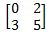# Linear Algebra: Tutoring Solution Final Exam

Free Practice Test Instructions:

Choose your answer to the question and click 'Continue' to see how you did. Then click 'Next Question' to answer the next question. When you have completed the free practice test, click 'View Results' to see your results. Good luck!

#### Question 2 2. What is the equation of the graph below?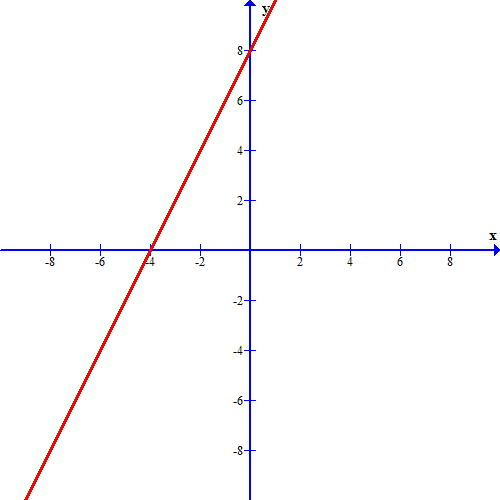#### Question 3 3. What is the determinant of the minor matrix related to the number 2 in the top row?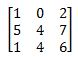#### Question 10 10. If the inverse of matrix A is this, what is the solution when the constants are 10 and 13?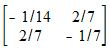#### Question 11 11. Which system of equations is represented by the following matrix? (Think of the vertical line as the equal sign.)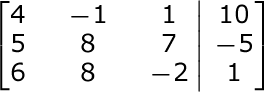#### Question 13 13. What is the determinant?#### Question 15 15. Find the determinant.